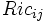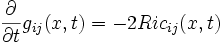# Flow method to prove existence of certain metrics

## Description

### Problem statement

We are given a differential manifold$M$, and we want to know whether there exists a Riemannian metric$g$ on$M$ such that$g$ satisfies a certain property of interest to us. This property could, for instance, be:

### Approach

The idea is as follows:

• Construct a differential operator on the space of sections of (0,2)-tensor fields such that the points which map to zero under that operator are precisely those satisfying the given property.
• Consider the flow equation associated with that differential operator. Note that this will be a vector flow equation, rather than a scalar one.
• Try to locate a trajectory for that flow equation for which the limit at infinity is well-defined.

## The Ricci-flat case

### The differential operator

A Ricci-flat metric is defined as a metric whose Ricci curvature is zero everywhere. Thus, a natural choice of differential operator is the Ricci curvature tensor$Ric_{ij}$ viewed as an operator that inputs the metric tensor and outputs the Ricci curvature tensor, both living in the space of (0,2)-tensor fields on the differential manifold.

However, for various reasons of normalization, the differential operator that we actually take is (-2) times the Ricci curvature tensor.

### The flow equation

The flow equation we get from the above is:$\frac{\partial}{\partial t} g_{ij}(x,t) = -2Ric_{ij} (x,t)$

This is termed the Ricci flow.

## The Einstein case

Fill this in later